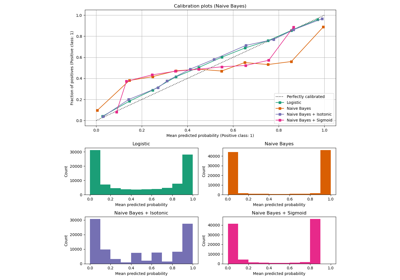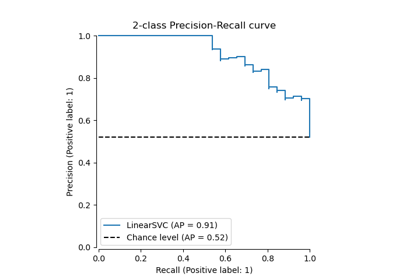# sklearn.metrics.precision_score¶

sklearn.metrics.precision_score(y_true, y_pred, labels=None, pos_label=1, average='binary', sample_weight=None, zero_division='warn')[source]

Compute the precision

The precision is the ratio tp / (tp + fp) where tp is the number of true positives and fp the number of false positives. The precision is intuitively the ability of the classifier not to label as positive a sample that is negative.

The best value is 1 and the worst value is 0.

Read more in the User Guide.

Parameters
y_true1d array-like, or label indicator array / sparse matrix

Ground truth (correct) target values.

y_pred1d array-like, or label indicator array / sparse matrix

Estimated targets as returned by a classifier.

labelslist, optional

The set of labels to include when average != 'binary', and their order if average is None. Labels present in the data can be excluded, for example to calculate a multiclass average ignoring a majority negative class, while labels not present in the data will result in 0 components in a macro average. For multilabel targets, labels are column indices. By default, all labels in y_true and y_pred are used in sorted order.

Changed in version 0.17: parameter labels improved for multiclass problem.

pos_labelstr or int, 1 by default

The class to report if average='binary' and the data is binary. If the data are multiclass or multilabel, this will be ignored; setting labels=[pos_label] and average != 'binary' will report scores for that label only.

averagestring, [None, ‘binary’ (default), ‘micro’, ‘macro’, ‘samples’, ‘weighted’]

This parameter is required for multiclass/multilabel targets. If None, the scores for each class are returned. Otherwise, this determines the type of averaging performed on the data:

'binary':

Only report results for the class specified by pos_label. This is applicable only if targets (y_{true,pred}) are binary.

'micro':

Calculate metrics globally by counting the total true positives, false negatives and false positives.

'macro':

Calculate metrics for each label, and find their unweighted mean. This does not take label imbalance into account.

'weighted':

Calculate metrics for each label, and find their average weighted by support (the number of true instances for each label). This alters ‘macro’ to account for label imbalance; it can result in an F-score that is not between precision and recall.

'samples':

Calculate metrics for each instance, and find their average (only meaningful for multilabel classification where this differs from accuracy_score).

sample_weightarray-like of shape (n_samples,), default=None

Sample weights.

zero_division“warn”, 0 or 1, default=”warn”

Sets the value to return when there is a zero division. If set to “warn”, this acts as 0, but warnings are also raised.

Returns
precisionfloat (if average is not None) or array of float, shape = [n_unique_labels]

Precision of the positive class in binary classification or weighted average of the precision of each class for the multiclass task.

Notes

When true positive + false positive == 0, precision returns 0 and raises UndefinedMetricWarning. This behavior can be modified with zero_division.

Examples

>>> from sklearn.metrics import precision_score
>>> y_true = [0, 1, 2, 0, 1, 2]
>>> y_pred = [0, 2, 1, 0, 0, 1]
>>> precision_score(y_true, y_pred, average='macro')
0.22...
>>> precision_score(y_true, y_pred, average='micro')
0.33...
>>> precision_score(y_true, y_pred, average='weighted')
0.22...
>>> precision_score(y_true, y_pred, average=None)
array([0.66..., 0.        , 0.        ])
>>> y_pred = [0, 0, 0, 0, 0, 0]
>>> precision_score(y_true, y_pred, average=None)
array([0.33..., 0.        , 0.        ])
>>> precision_score(y_true, y_pred, average=None, zero_division=1)
array([0.33..., 1.        , 1.        ])


## Examples using sklearn.metrics.precision_score¶